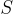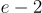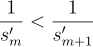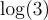## Sunday, June 18, 2023

### "Arithmetic Density and Related Concepts"

I. Counting Arithmetic Progressions

and

#### II. Relative Arithmetic Density

There are two other uses for our counting function over the positive integers which will be discussed in both this section as well as the following section. The first application involves computing the relative arithmetic density of our sequence, but before we begin it is necessary to elaborate on the definition of partial densities.

which is just some real number less than or equal to one.

#### III. Natural Arithmetic Density

The second use for our AP counting functions involves a more naturalistic interpretation of arithmetic density, as far as real number valuations are concerned. We can arrive at such a definition by taking advantage of the fact that the counting function for any infinite progression has maximal growth. It then becomes an arbitrary choice of which complementary sequence we might use to construct a suitable infinite series for our valuation. The author has chosen the sequence of factorials because firstly they converge for all AP counting functions, and secondly because they allow for more convenient algebraic analysis. The formula for the natural arithmetic density ofis thus defined

This series is approximately 1.42638919894259387857 when the counting function is taken over any infinite progression. For the rare case when there is only one progression of length three at the beginning of the given sequence, the series above is equal to.

IV. The Partitional Convergence Theorem

(i) Proof of the theorem.

Assuming the infinite series

and

In order to establish our proof, let us first draw our attention to the terminal values at the end of each finite series. Without the addition of these single terms, any particular series falls short of those before it according to the second inequality above. Therefore, if all of the finite series are to diverge from any particular constant, that would imply each terminal value is greater than the previous one, or. This cannot be the case since our infinite subsequence is monotonically increasing, and so its reciprocal elements must tend toward zero. As an example of the proof, let it be noted that the divergence constant for the positive integers is.

and

(ii) Alternative representation of divergent series.

A further consequence of the theorem is that partial sums of divergent series can now be represented using the divergence constant mentioned above. To do so requires the introduction of an error function which adjusts the constant according to the interval over which the function is taken, i.e.

which implies

REFERENCES

  Weisstein, Eric W. "Arithmetic Progression." From Mathworld--A Wolfram web resource. (http://mathworld.wolfram.com/ArithmeticProgression.html)

  Entry A330285 in The On-Line Encyclopedia of Integer Sequences,

  Entry A051336 in The On-Line Encyclopedia of Integer Sequences, (http://oeis.org/A051336)

  Weisstein, Eric W. "q-Polygamma Function." From Mathworld--A Wolfram web resource. (https://mathworld.wolfram.com/q-PolygammaFunction)

  Weisstein, Eric W. "Backward Difference." From Mathworld--A Wolfram web resource. (http://mathworld.wolfram.com/BackwardDifference)

### "Arithmetic Density and Related Concepts"

I. Counting Arithmetic Progressions Given an arbitrary increasing sequence of positive integers there is associated with it, a counting...